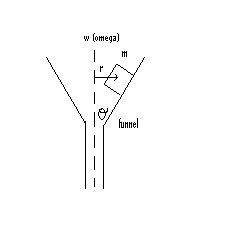# Friction problem on cube of mass

a very small cube of mass m is placed on the inside wall of a funnel. The wall of the funnel makes an angle theta with the vertical axis of rotation (dotted line). The center of the cube is a distance r from the axis of rotation. the cube is held by static friciton. The funnel is then rotated about a vertical axis at an angular speed of w (omega). it is found that the angular speed can be increased to a maximum value w(max) (omega max) at which point the cube is no longer held by the static frictional force and it flies out of the funnel. calculate w(max)i separated everything into x and y components, but i think i did this wrong
i got equations
Fstatic - Fg cos theta = mw(max)^2r
Fn - Fg sin theta = ma
am i suppose to set a to zero? and are those even right to begin with

Last edited:

## Answers and Replies

okay, this is what i got so far, i dont know if its right. correct me if i had a mistake

taking xaxis is // to side of funnel, and yais is perpendicular to side of funnel, i drew fbd and the forces i got are
x components : Fs, - mg cos theta
y components : Fn, - mg sin theta

so i hvae two equations:
Fs - mg cos theta = mw^2r (1)
Fn - mg sin theta = ma
Fn - mg sin theta = 0
Fn = mg sin theta
since mew (u) = Fs/Fn
Fs = u Fn
Fs = u mg sin theta
subbign that into equation (1)
u mg sin theta - mg cos theta = mw^2r
masses cancel
u g sin theta - g cos theta = w^2r
isolating for w:
w = sqrt [g((u sin theta + cos theta)/r)]

is that right?

andrevdh
Homework Helper
I dont think so. Your Fs should be negative, but even more serious is that the centripetal acceleration of the cube is horizontal, pointing inwards towards the vertical axis.